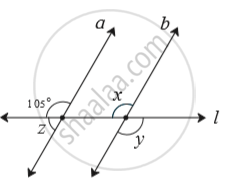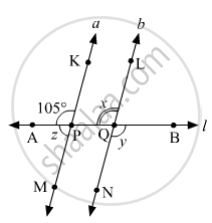SSC (English Medium) Class 8Maharashtra State Board
Share

# In the Adjoining Figure, Line a || Line B. Line L is a Transversal. Find the Measures of ∠X, ∠Y, ∠Z Using the Given Information. - SSC (English Medium) Class 8 - Mathematics

ConceptProperties of Angles Formed by Two Parallel Lines and a Transversal

#### Question

In the adjoining figure, line a || line b.  line l is a transversal. Find the measures of ∠x, ∠y, ∠z using   the given information.#### SolutionLet us mark the points A and B on l; K and M on a; L and N on b.
Suppose KM and LN intersect AB at P and Q respectively.
Since, a||b and l is a transversal, then
m∠PQL = m∠APK    (Corresponding angles)
⇒ x = 105°
Since, AB and LN are straight lines that intersect at Q, then
m∠BQN = m∠PQL    (Vertically opposite angles)
⇒ y = x
⇒ y = 105°
Since, AB is a straight line and ray QN stands on it, then
m∠BQN + m∠PQN = 180°    (Angles in linear pair)
⇒ y + m∠PQN = 180°
⇒ 105° + m∠PQN = 180°
⇒ m∠PQN = 180° − 105°
⇒ m∠PQN = 75°
Now, m∠APM = m∠PQN    (Corresponding angles)
⇒ z = 75°

Is there an error in this question or solution?

#### APPEARS IN

Balbharati Solution for Balbharati Class 8 Mathematics (2019 to Current)
Chapter 2: Parallel lines and transversals
Practice Set 2.2 | Q: 4 | Page no. 12
Solution In the Adjoining Figure, Line a || Line B. Line L is a Transversal. Find the Measures of ∠X, ∠Y, ∠Z Using the Given Information. Concept: Properties of Angles Formed by Two Parallel Lines and a Transversal.
S